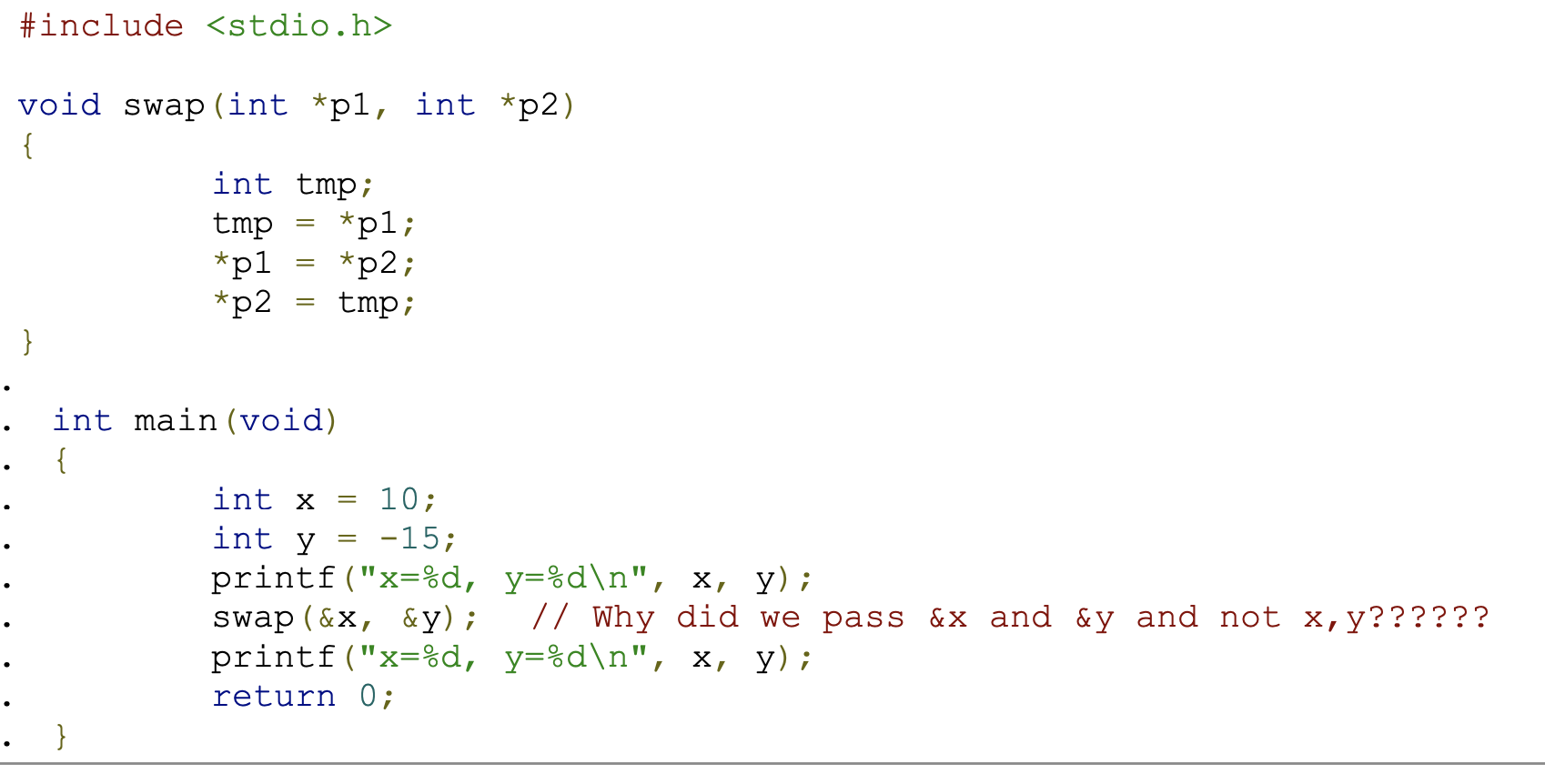# Pointer §

This is the most confusing topic ever. Check out the Wikipedia to fully understand https://en.wikipedia.org/wiki/Pointer_(computer_programming)

A pointer points to an address of another variable.

C++ Pointer Syntax

In CS137, the syntax we learned to declare a pointer is int *a. However, in general, the preferred syntax in C++ is int* a. Do that at interviews and in practice.

string* mystring; // Preferred
string *mystring;
string * mystring;

An Array name is just a pointer to the first element in the array, i.e. if a is an array,

printf("%p\n", a);
printf("%p\n", &a);

print the same thing (will print the address).

int i = 6;
int *p; //It's important to declare what type the pointer is point at
p = &i; //the & in front gives the memory address of i
*p = 10; //Putting the * will change the value. In this case, p is changed to 10, so i changes to 10 as well

printf("%d \n", i); //Will print 10

int *q = p;
*q = 17;
printf("%d \n", i); //Will print 17

The asterisks can be confusing.

• * for multiplication
• * to define pointer
• * as a dereferencing operator.

Pointers can be super helpful in certain scenarios. Consider the case where you want to create a function swap(x,y). If you don’t pass the address, it will use a copy of the value and not actually swap x,y. You need to pass the addresses of x,y, and in the implementation, use pointers as arguments of the function.Note

Since C only supports Call by Value, what we are actually doing above is using a pointer to “cheat” and reference the variables when the function is called.

### Pointer Arithmetic §

We can actually add integers to pointers. It just moves the pointer to the next address.

int a = { 2, 3, 4, 5, 6, 7, 8, 9 };
int *p, *q, i;
p = &(a); // p points to a
q = p + 3;// q points to a
p += 4;// p points to a
q = q - 2; // q points to a

//This is also legal
i = p - q; // i = 3
i = q - p; // i = -3

The * operator and ++ operator can be combined:

• *p++ is the same as *(p++) (Use *p first then increment pointer).
• (*p)++ (Use *p first then increment *p, increment the content that p is pointing at).
• *++p or *(++p) (Increment p first then use *p after increment).
• ++*p or ++(*p) (Increment *p first then use *p after increment).

### Void Pointer §

Void pointers can point to any data type. Used in Map and Reduce.

You need to cast the type like (int *)pointer to cast it.

### Null Pointer §

Since pointers are memory addresses, we need to be able to distinguish from a pointer to something and a pointer to nothing. The NULL pointer is how we do this. It can be called by

int *p = NULL;

For C++, Use nullptr to say that a pointer is pointing to nothing.

int *p = nullptr;

Note: From what I remember, there is some subtle difference in the way NULL and nullptr is used for C and C++.

### WARNING BUG §

I ran into this bug with multi-line pointer declaration.

int a, b, c; // This is fine

However,

int* a, b, c; // INCORRECT

// Equivalent to
int *a;
int b;
int c;

### Pointer vs. Pointer Reference §

Ran into this while working on my Biquadris project.

This blew my mind, but int*& x is allowed in C++. It declares a reference to a pointer to an integer. Essentially, it allows you to create an alias for a pointer variable.

void modifyPointer(int*& ptr) {
ptr = new int(10); // Modifies the original pointer
}

int main() {
int* myPointer = nullptr;
modifyPointer(myPointer); // myPointer now points to a new int with value 10
delete myPointer; // Clean up the allocated memory
return 0;
}

Also see unique_ptr, where I try to understand the different between unique_ptr<Level> and unique_ptr<level>&.

Can you have a pointer to a Reference?

NO! Remember that a reference is just an alias. A pointer is a place in memory that has the address of something else, but a reference is NOT. StackOverflow